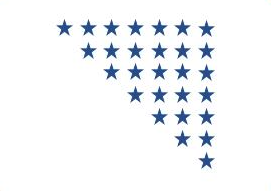# C Program to Print Reversed Mirrored Right Triangle Star Pattern

Here is a C program to print reversed inverted right triangle star (*) pattern of n rows using for loop. For a reversed mirrored right triangle star pattern of 7 rows. Program's output should be:#### Required Knowledge

Algorithm to print reversed mirrored right triangle star pattern using loop
If you look closely, this pattern is similar to inverted right triangle star pattern. For Rth row, we have to print R space characters before stars.
• Take the number of rows(N) of reversed inverted right triangle as input from user using scanf function.
• Each row(R) contains N characters, R space characters followed by N-R star (*) character.
• We will use two for loops. Outer for loop will print one row in one iteration.
• One iteration of inner loop will first print R space characters followed by N-R star (*) character

Here is the matrix representation of the mirrored triangle star pattern. The row numbers are represented by i whereas column numbers are represented by j.## C program to print reversed mirrired right triangle star pattern

```#include <stdio.h>

int main() {
int i, j, rows;

printf("Enter the number of rows\n");
scanf("%d", &rows);

for (i = 0; i < rows; i++) {
for (j = 0; j < rows; j++) {
if (j < i) {
printf(" ");
} else {
printf("*");
}
}
printf("\n");
}
return 0;
}
```
Output
```Enter the number of rows
6
******
*****
****
***
**
*
```

Related Topics
 C program inverted right triangle pattern C program right triangle star pattern C program reversed right triangle star pattern C program natural number triangle pattern C program palindrome triangle pattern C program same row element triangle pattern C program prime number triangle pattern C program rhombus star pattern C program diamond star pattern List of all C pattern programs